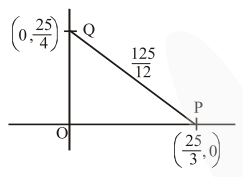# Let the tangent to the circle

Question:

Let the tangent to the circle $x^{2}+y^{2}=25$ at the point $R(3,4)$ meet $x$-axis and $y$-axis at point $P$ and $\mathrm{Q}$, respectively. If $\mathrm{r}$ is the radius of the circle passing through the origin $\mathrm{O}$ and having centre at the incentre of the triangle OPQ, then $\mathrm{r}^{2}$ is equal to

1. $\frac{529}{64}$

2. $\frac{125}{72}$

3. $\frac{625}{72}$

4. $\frac{585}{66}$

Correct Option: , 3

Solution:

Tangent to circle $3 x+4 y=25$$\mathrm{OP}+\mathrm{OQ}+\mathrm{OR}=25$

Incentre $=\left(\frac{\frac{25}{4} \times \frac{25}{3}}{25}, \frac{\frac{25}{4} \times \frac{25}{3}}{25}\right)$

$=\left(\frac{25}{12}, \frac{25}{12}\right)$

$\therefore r^{2}=2\left(\frac{25}{12}\right)^{2}=2 \times \frac{625}{144}=\frac{625}{72}$